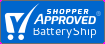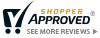Save 5% Extra Online. Use code: SAV121MenuSave 5% Extra Online. Use code: SAV121More Battery Answers

What are volts

Volts - or V - is an electrical measurement of energy potential. Mathematically voltage is commonly measured by V= I x R; where V=Voltage, I=Current, R=Resistance.

Voltage can also be defined as Electrical Potential difference - a quantity in physics related to the amount of energy that would be required to move an object from one place to another against various types of force. In the fields of electronics the electrical potential difference is the amount of work per charge needed to move electric charge from the second point to the first, or equivalently, the amount of work that unit charge flowing from the first point to the second can perform. A battery contains four unique types of voltage measurements. Each voltage measurement type residing in a battery effects battery life.
1. Float Voltage  is battery voltage at zero current (with battery disconnected).
2. Nominal Voltage  is battery voltage range 3.7V, 5.2V, 10.2V, 12V etc that says that a voltage range exists depending on the number of cells in the battery. For example a 12 Volt battery is made of 6 cells and has a Float voltage of about 12V.
3. Charge Voltage - The voltage of a battery while charging.
4. Discharge Voltage - The voltage of a battery while discharging. Again, this voltage is determined by the charge state and the current flowing in the battery.